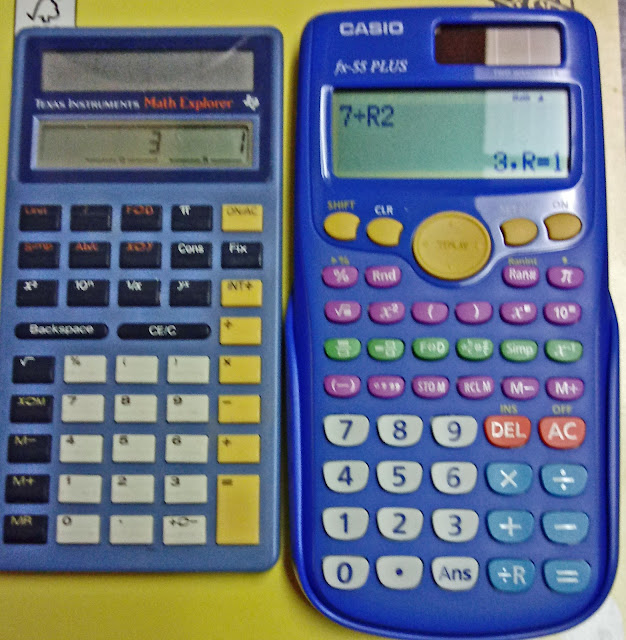## Wednesday, August 15, 2018

### Review: Casio fx-55 Plus

Review:  Casio fx-55 Plus

General Information

Company:  Casio
Type:  Fractions, Elementary Math
Memory:  1
Battery:  Solar with battery backup (1 LR44)
Years:  2012 - current
Cost:  \$10.00 - \$20.00, I paid \$12.99
Operating Logic:  Algebraic

Target Audience:

* Elementary and middle school students
* Anyone who wants a few more functions than the regular four-function calculator, but not a scientific calculator
* You want a calculator with an emphasis on fractions

Features

The major emphasis on the Casio fx-55 Plus is on fractions.  We’ll discuss this in the next section in detail.

The screen has a textbook display, meaning fractions and all other calculations are displayed as you would write them.  Fractions are simplified.  If MathO (Math Output) is selected, square roots and terms of pi (π) shown in exact form.  If LineO is selected, all answers (except fractions) are shown in decimal form.  No matter what the display mode is, you can always decimal approximation by pressing [SHIFT] [ = ].

Example (MathO):

12 returns 2 3

5^2 * π returns 25π

You enter expressions the way you would write them with the Casio fx-55 Plus.  The display is a mutli-line display, the expression on the top of the screen and the answer is on the bottom of the screen.

The keyboard is simple where only four keys have shifted functions.  The OFF function is shifted as well.

Other functions include reciprocal, random numbers, including random integers, and calculations in Degrees-Minutes-Seconds [ ° ‘ “ ].

The memory has four dedicated keys: store, recall, add to memory (M+), and subtract from memory (M-).

The percent keys work a bit differently from the normal four-function calculators (and is not very intuitive).

[%] divides the argument by 100 and that’s it.  Its fine when you are working with multiplying or dividing percentages, but adding and subtracting percent will take an algorithm:

X + Y%:  enter X + X * Y %
X – Y%:  enter X – X * Y %

[>%] multiplies the result by 100 and displays the answer in percentage notation.

Example:  3 [ = ] [ SHIFT ] (>%) returns 300%

Let’s Talk Fractions

The fraction keys are marked lime green on the Casio fx-55 Plus.  Let’s go over the keys in detail:

[ []/[] ] (1st key from the left):  fraction template

[ [] []/[] ] (2nd key from the left):  mixed number template

[ F←→D] (3rd key from the left): switches the result between exact (fractions, exact square roots, terms of π) and decimal answer

[ a b/c ←→ d/c ]:  (4th key from the left)

Manual Simplified mode:  This key does nothing.

Auto Simplified mode: This key converts the result between improper and proper fraction notation.

[ Simp ]:  calls the simplify conversion to the entry.  In Manual Simplified mode, any fraction that is not reduced to its irreducible form will have a down arrow indicator ( ).  Also executing Simp in Manual Simplified mode not only returns the reduced fraction, but the factor used into reducing such fraction.

[x^-1]:  The reciprocal function

I also want to point a very handy key, the remainder division function ( [÷R]), which is located on the bottom right hand corner of the keyboard.  This function returns both the quotient and remainder.

Example:  77 ÷R 13 returns 5, R = 12

Only the quotient is stored in last answer (Ans).

Let’s compare this Casio fx-55 Plus with an older fraction oriented calculator, the Texas Instruments Math Explorer (aka TI-12).

Comparison:  Casio fx-55 Plus (2012-present) vs. Texas Instruments Math Explorer (1978-1998)TI Math Explorer Casio fx-55 Plus 1 line display Multi-line, textbook display No shifted functions 4 shifted functions N/A Degrees-Minutes Seconds calculations, random numbers and integers Constant arithmetic storage function through the [ Cons ] key. N/A 10^n is limited to integers 0-7 10^x allows for all real numbers between up to, but not including, 100.  Used to work with numbers in scientific notation Int÷ works with only positive integers ÷R works with real, positive numbers (any non-positive numbers makes ÷R work like ÷)

Verdict

I like this calculator for middle school and elementary students. The Casio fx-55 Plus presents a few extra functions but without the many scientific functions that may intimidate some students.  (emphasis on some)  I wish the percent would work like we would write expressions when it comes to addition or subtraction (i.e. X + Y% instead of X + X * Y%), but this is understandable.  I also wish the proper/improper toggle worked in Manual Simplified mode.

The display is great because the user can see the expression and answer at the same time.  I really like that this calculator also returns exact answers in calculations involving square roots and π.

I can see this calculator being used beyond the classroom, especially in everyday basic math calculations.

In any case, the Casio fx-55 Plus serves a great introductory calculator.

Eddie

All original content copyright, © 2011-2018.  Edward Shore.   Unauthorized use and/or unauthorized distribution for commercial purposes without express and written permission from the author is strictly prohibited.  This blog entry may be distributed for noncommercial purposes, provided that full credit is given to the author.  Please contact the author if you have questions.